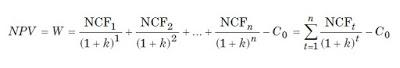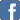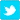# Time Value For Money, Risk Premimum, Interest Rate, Capital Recovery

By Renu Kumari
Time Value for Money : - Individual investors generally prefer possession of a given amount of cash now, rather than the same amount at some future time. This time preference for money may arise because of(a) uncertainty of cash flows,(b) subjective preference for consumption, and(c) availability of investment opportunities.The last reason is the most sensible justification for the time value of money

Risk Premium: - Interest rate demanded, over and above the risk-free rate as compensation for time, to account for the uncertainty of cash flows.Interest Rate or Time Preference Rate: - Rate which gives money its value, and facilitates the comparison of cash flows occurring at different time periods.Required Interest Rate: - A risk-premium rate is added to the risk- free time preference rate to derive required interest rate from risky investments.Compounding: - It means calculating future values of cash flows at a given interest rate at the end of a given period of time.Future Value (F) of a Lump Sum: -  Today (P) for periods at rate of interest is given by the following formula:The compound value factor, CVFn ,i can be found out from Table A given at the end of the book.

Future Value of an Annuity: - that is, the same amount of cash each year for periods at rate of interest is given by the following equationThe compound value of an annuity factor (CVAFn,i) can be found out from Table B given in Annexure at the end of the book.
Sinking Fund: -  An annuity to be deposited for periods at rate of interest to accumulate to a given sum. The following equation can be used:Sinking Fund factor (SFFn, i) is a reciprocal of CVAFn, i.
Discounting: - It means calculating the present value of cash flows at a given interest rate at the beginning of a given period of time.
Present Value (P) of a Lump Sum (F): - It occured at the end of period at rate of interest is given by the following equation:The present value factor (PVFn, i) can be obtained from Table C given in Annexure at the end of the book.
Present Value of an Annuity (A) occurring for periods at rate of interest can be found out as follows:Table can be used to find out the present value of annuity factor (PVAFn, i).

Capital Recovery: - It determined annual cash flows to be earned to recover a given investment. The following equation can be used:Notice that the capital recovery factor (CRFn,i) is a reciprocal of the present valueannuity factor, PVAFn, i.
Wealth or Net Present Value: - It is defined as the difference between the present value of cash inflows (benefits) and the present value of cash outflows (costs). Wealth maximisation principle uses interest rate to find out the present value of benefits and costs, and as such, it considers their timing and risk. The following formula can be used to calculate NPV or wealth of any pattern of cashflows:Multi-period Compounding:  When interest compounds for more than once in a given period of time, it is called multi-period compounding. If is the nominal interest rate for a period, the effective interest rate (EIR) will be more than the nominal rate in multi-period compounding since interest on interest within ayear will also be earned, EIR is given as follows:where is the number of compounding in a year and is number of years.
Internal Rate of Return (IRR):  IRR is the rate, which equates the present value of cash flows to the initial investment. Thus in operational terms, in the present value equation, all variables are known except rcan be found out by trial and error method: